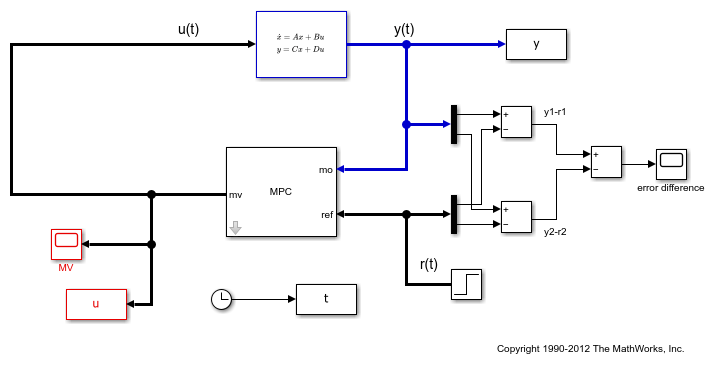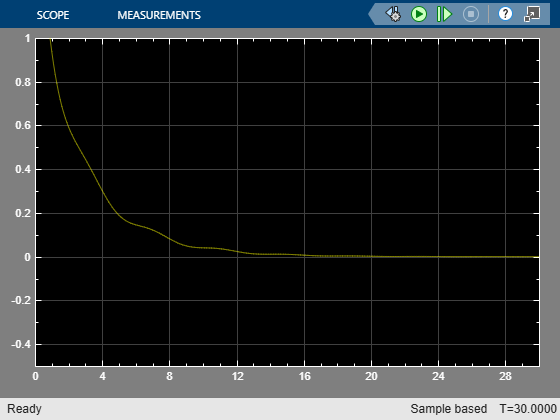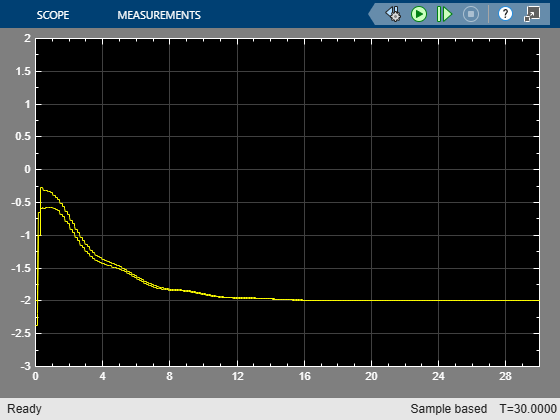# Specifying Alternative Cost Function with Off-Diagonal Weight Matrices

This example shows how to use non-diagonal weight matrices in a model predictive controller.

### Define Plant Model and MPC Controller

The linear plant model has two inputs and two outputs.

```plant = ss(tf({1,1;1,2},{[1 .5 1],[.7 .5 1];[1 .4 2],[1 2]})); [A,B,C,D] = ssdata(plant); Ts = 0.1; % sampling time plant = c2d(plant,Ts); % convert to discrete time ```

Create MPC controller.

```p=20; % prediction horizon m=2; % control horizon mpcobj = mpc(plant,Ts,p,m); ```
```-->The "Weights.ManipulatedVariables" property of "mpc" object is empty. Assuming default 0.00000. -->The "Weights.ManipulatedVariablesRate" property of "mpc" object is empty. Assuming default 0.10000. -->The "Weights.OutputVariables" property of "mpc" object is empty. Assuming default 1.00000. ```

Define constraints on the manipulated variable.

```mpcobj.MV = struct('Min',{-3;-2},'Max',{3;2},'RateMin',{-100;-100},'RateMax',{100;100}); ```

Define non-diagonal output weight. Note that it is specified inside a cell array.

```OW = [1 -1]'*[1 -1]; % Non-diagonal output weight, corresponding to ((y1-r1)-(y2-r2))^2 mpcobj.Weights.OutputVariables = {OW}; % Non-diagonal input weight, corresponding to (u1-u2)^2 mpcobj.Weights.ManipulatedVariables = {0.5*OW}; ```

### Simulate Using SIM Command

Specify simulation options.

```Tstop = 30; % simulation time Tf = round(Tstop/Ts); % number of simulation steps r = ones(Tf,1)*[1 2]; % reference trajectory ```

Run the closed-loop simulation and plot results.

```[y,t,u] = sim(mpcobj,Tf,r); subplot(211) plot(t,y(:,1)-r(1,1)-y(:,2)+r(1,2));grid title('(y_1-r_1)-(y_2-r_2)'); subplot(212) plot(t,u);grid title('u'); ```
```-->Assuming output disturbance added to measured output channel #1 is integrated white noise. -->Assuming output disturbance added to measured output channel #2 is integrated white noise. -->The "Model.Noise" property of the "mpc" object is empty. Assuming white noise on each measured output channel. ```To run this example, Simulink® is required.

```if ~mpcchecktoolboxinstalled('simulink') disp('Simulink(R) is required to run this part of the example.') return end ```

Now simulate closed-loop MPC in Simulink®.

```mdl = 'mpc_weightsdemo'; open_system(mdl); sim(mdl) ``````bdclose(mdl); ```